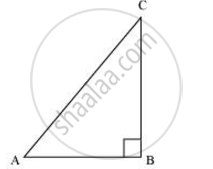# If sin A =3/4 , calculate cos A and tan A. - Mathematics

If sin A =3/4 , calculate cos A and tan A.

#### Solution

Let ΔABC be a right-angled triangle, right-angled at point B.Given that,

sin A = 3/4

(BC)/(AC) = 3/4

Let BC be 3k. Therefore, AC will be 4k, where k is a positive integer.

Applying Pythagoras theorem in ΔABC, we obtain

AC2 = AB2 + BC2

(4k)2 = AB2 + (3k)2

16k 2 − 9k 2 = AB2

7k 2 = AB2

AB = sqrt7k

cos A = ("Side adjacent to"angleA)/"Hypotenuse"

=(AB)/(AC) = sqrt(7k)/(4k) = sqrt7/4

tan A = ("Side adjacent to"angleA)/("Side adjacent to"angleA)

= (BC)/(AB) = (3k)/(sqrt7k) = 3/sqrt7

Concept: Trigonometric Ratios
Is there an error in this question or solution?
Chapter 8: Introduction to Trigonometry - Exercise 8.1 [Page 181]

#### APPEARS IN

NCERT Class 10 Maths
Chapter 8 Introduction to Trigonometry
Exercise 8.1 | Q 3 | Page 181
Share